CBSE (Science) Class 11CBSE
Share

# HC Verma solutions for Class 11 Physics chapter 16 - Sound Waves

#### HC Verma Concept of Physics Part-1 (2018-2019 Session) by H.C Verma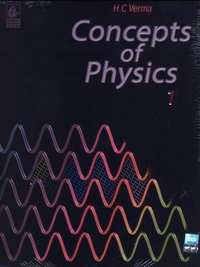## Chapter 16: Sound Waves

#### HC Verma solutions for Class 11 Physics Chapter 16 Exercise Short Answers [Page 351]

Short Answers | Q 1 | Page 351

If you are walking on the moon, can you hear the sound of stones cracking behind you? Can you hear the sound of your own footsteps?

Short Answers | Q 2 | Page 351

Can you hear your own words if you are standing in a perfect vacuum? Can you hear your friend in the same conditions?

Short Answers | Q 3 | Page 351

A vertical rod is hit at one end. What kind of wave propagates in the rod if (a) the hit is made vertically (b) the hit is made horizontally?

Short Answers | Q 4 | Page 351

Two loudspeakers are arranged facing each other at some distance. Will a person standing behind one of the loudspeakers clearly hear the sound of the other loudspeaker or the clarity will be seriously damaged because of the 'collision' of the two sounds in between?

Short Answers | Q 5 | Page 351

The voice of a person, who has inhaled helium, has a remarkably high pitch. Explain on the basis of resonant  vibration of vocal cord filled with air and with helium.

Short Answers | Q 6 | Page 351

Draw a diagram to show the standing pressure wave and standing displacement wave for the 3rd overtone mode of vibration of an open organ pipe.

Short Answers | Q 7 | Page 351

Two tuning forks vibrate with the same amplitude but the frequency of the first is double the frequency of the second. Which fork produces more intense sound in air?

Short Answers | Q 8 | Page 351

In discussing Doppler effect, we use the word "apparent frequency". Does it mean that the frequency of the sound is still that of the source and it is some physiological phenomenon in the listener's ear that gives rise to Doppler effect? Think for the observer approaching the source and for the source approaching the observer.

#### HC Verma solutions for Class 11 Physics Chapter 16 Exercise MCQ [Pages 351 - 352]

MCQ | Q 1 | Page 351

Consider the following statements about sound passing through a gas.
(A) The pressure of the gas at a point oscillates in time.
(B) The position of a small layer of the gas oscillates in time.

• Both A and B are correct.

• A is correct but B is wrong.

• B is correct but A is wrong.

• Both A and B are wrong.

MCQ | Q 2 | Page 351

When we clap our hands, the sound produced is best described by Here p denotes the change in pressure from the equilibrium value.

• $p = p_0 \sin\left( kx - \omega t \right)$

• $p = p_0 \sin kx \cos \omega t$

• $p = p_0 \cos kx \sin \omega t$

• $p = \sum p_{0n} \sin \left( k_n x - \omega_n t \right)$

MCQ | Q 3 | Page 351

The bulk modulus and the density of water are greater than those of air. With this much of information, we can say that velocity of sound in air

• is larger than its value in water

• is smaller than its value in water

•  is equal to its value in water

• cannot be compared with its value in water.

MCQ | Q 4 | Page 351

A tuning fork sends sound waves in air. If the temperature of the air increases, which of the following parameters will change?

• Displacement amplitude

• Frequency

• Wavelength

• Wavelength

MCQ | Q 5 | Page 351

When sound wave is refracted from air to water, which of the following will remain unchanged?

• Wave number

• Wavelength

• Wave velocity

• Frequency

MCQ | Q 6 | Page 351

The speed of sound in a medium depends on

• the elastic property but not on the inertia property

• the inertia property but not on the elastic property

• the elastic property as well as the inertia property

• neither the elastic property nor the inertia property.

MCQ | Q 7 | Page 351

Two sound waves move in the same direction in the same medium. The pressure amplitudes of the waves are equal but the wavelength of the first wave is double the second. Let the average power transmitted across a cross section by the first wave be P1 and that by the second wave be P2. Then

• $P_1 = P_2$

• $P_1 = 4 P_2$

• $P_2 = 2 P_1$

• $P_2 = 4P .$

MCQ | Q 8 | Page 351

When two waves with same frequency and constant phase difference interfere,

•  there is a gain of energy

• there is a loss of energy

• the energy is redistributed and the distribution changes with time

• the energy is redistributed and the distribution remains constant in time.

MCQ | Q 9 | Page 352

An open organ pipe of length L vibrates in its fundamental mode. The pressure variation is maximum

• at the two ends

• at the middle of the pipe

• at distance L/4 inside the ends

• at distances L/8 inside the ends.

MCQ | Q 10 | Page 352

An organ pipe, open at both ends, contains

• longitudinal stationary waves

• longitudinal travelling waves

• transverse stationary waves

• transverse travelling waves.

MCQ | Q 11 | Page 352

A cylindrical tube, open at both ends, has a fundamental frequency v. The tube is dipped vertically in water so that half of its length is inside the water. The new fundamental frequency is

• v/4

• v/2

•  v

• 2v

MCQ | Q 12 | Page 352

The phenomenon of beats can take place

• for longitudinal waves only

•  transverse waves only

• for both longitudinal and transverse waves

• for sound waves only

MCQ | Q 13 | Page 352

A tuning fork of frequency 512 Hz is vibrated with a sonometer wire and 6 beats per second are heard. The beat frequency reduces if the tension in the string is slightly increased. The original frequency of vibration of the string is

• 506 Hz

• 512 Hz

• 518 Hz

• 524 Hz.

MCQ | Q 14 | Page 352

The engine of a train sounds a whistle at frequency v. The frequency heard by a passenger is

• $> v$

• $< v$

• $= \frac{1}{v}$

• $= v$

MCQ | Q 15 | Page 352

The change in frequency due to Doppler effect does not depend on

• the speed of the source

• the speed of the observer

• the frequency of the source

• separation between the source and the observer.

MCQ | Q 16 | Page 352

A small source of sounds moves on a circle as shown in figure and an observer is sitting at O. Let $v_1, v_2, v_3$ be the frequencies heard when the source is at A, B and C respectively.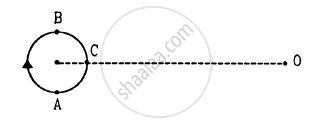• $v_1 > v_2 > v_3$

• $v_1 = v_2 > v_3$

• $v_2 > v_3 > v_1$

• $v_1 > v_3 > v_2$

#### HC Verma solutions for Class 11 Physics Chapter 16 Exercise MCQ [Page 352]

MCQ | Q 1 | Page 352

When you speak to your friend, which of the following parameters have a unique value in the sound produced?

• Frequency

• Wavelength

• Amplitude

• Wave velocity

MCQ | Q 2 | Page 352

An electrically maintained tuning fork vibrates with constant frequency and constant amplitude. If the temperature of the surrounding air increases but pressure remains constant, the produced will have

(a) larger wavelength
(b) larger frequency
(c) larger velocity
(d) larger time period.

MCQ | Q 3 | Page 352

The fundamental frequency of a vibrating organ pipe is 200 Hz.

(a) The first overtone is 400 Hz.
(b) The first overtone may be 400 Hz.
(c) The first overtone may be 600 Hz.
(d) 600 Hz is an overtone.

MCQ | Q 4 | Page 352

A source of sound moves towards an observer.

• The frequency of the source is increased.

• The velocity of sound in the medium is increased.

• The wavelength of sound in the medium towards the observer is decreased.

• The amplitude of vibration of the particles is increased.

MCQ | Q 5 | Page 352

A listener is at rest with respect to the source of sound. A wind starts blowing along the line joining the source and the observer. Which of the following quantities do not change?
(a) Frequency
(b) Velocity of sound
(c) Wavelength
(d) Time period

#### HC Verma solutions for Class 11 Physics Chapter 16 [Pages 352 - 357]

Q 1 | Page 352

A steel tube of length 1.00 m is struck at one end. A person with his ear closed to the other end hears the sound of the blow twice, one travelling through the body of the tube and the other through the air in the tube. Find the time gap between the two hearings. Use the table in the text for speeds of sound in various substances.

Q 2 | Page 353

At a prayer meeting, the disciples sing JAI-RAM JAI-RAM. The sound amplified by a loudspeaker comes back after reflection from a building at a distance of 80 m from the meeting. What maximum time interval can be kept between one JAI-RAM and the next JAI-RAM so that the echo does not disturb a listener sitting in the meeting. Speed of sound in air is 320 m s−1.

Q 3 | Page 353

A man stands before a large wall at a distance of 50.0 m and claps his hands at regular intervals. Initially, the interval is large. He gradually reduces the interval and fixes it at a value when the echo of a clap merges every 3 seconds, find the velocity of sound in air.

Q 4 | Page 353

A person can hear sound waves in the frequency range 20 Hz to 20 kHz. Find the minimum and the maximum wavelengths of sound that is audible to the person. The speed of sound is 360 m s−1.

Q 5 | Page 353

Find the minimum and maximum wavelengths of sound in water that is in the audible range (20−20000 Hz) for an average human ear. Speed of sound in water = 1450 m s−1.

Q 6 | Page 353

Sound waves from a loudspeaker spread nearly uniformly in all directions if the wavelength of the sound is much larger than the diameter of the loudspeaker. (a)Calculate the frequency for which the wavelength of sound in air is ten times the diameter of the speaker if the diameter is 20 cm. (b) Sound is essentially transmitted in the forward direction if the wavelength is much shorter than the diameter of the speaker. Calculate the frequency at which the wavelength of the sound is one tenth of the diameter of the speaker described above. Take the speed of sound to be 340 m/s.

Q 7 | Page 353

Ultrasonic waves of frequency 4.5 MHz are used to detect tumour in soft tissue. The speed of sound in tissue is 1.5 km s−1 and that in air is 340 m s−1. Find the wavelength of this ultrasonic wave in air and in tissue.

Q 8 | Page 353

The equation of a travelling sound wave is y = 6.0 sin (600 t − 1.8 x) where y is measured in 10−5 m, t in second and x in metre. (a) Find the ratio of the displacement amplitude of the particles to the wavelength of the wave. (b) Find the ratio of the velocity amplitude of the particles to the wave speed.

Q 9 | Page 353

A sound wave frequency 100 Hz is travelling in air. The speed of sound in air is 350 m s−1. (a) By how much is the phase changed at a given point in 2.5 ms? (b) What is the phase difference at a given instant between two points separated by a distance of 10.0 cm along the direction of propagation?

Q 10 | Page 353

Two point sources of sound are kept at a separation of 10 cm. They vibrate in phase to produce waves of wavelength 5.0 cm.  What would be the phase difference between the two waves arriving at a point 20 cm from one source (a) on the line joining the sources and (b) on the perpendicular bisector of the line joining the sources?

Q 11 | Page 353

Calculate the speed of sound in oxygen from the following data. The mass of 22.4 litre of oxygen at STP (T = 273 K and p = 1.0 × 105 N m−2) is 32 g, the molar heat capacity of oxygen at constant volume is Cv = 2.5 R and that at constant pressure is Cp = 3.5 R.

Q 12 | Page 353

The speed of sound as measured by a student in the laboratory on a winter day is 340 m s−1 when the room temperature is C17°. What speed will be measured by another student repeating the experiment on a day when the room temperature is 32°C?

Q 13 | Page 353

At what temperature will the speed of sound be double of its value at 0°C?

Q 14 | Page 353

The absolute temperature of air in a region linearly increases from T1 to T2 in a space of width d. Find the time taken by a sound wave to go through the region in terms of T1T2d and the speed v of sound at 273 K. Evaluate this time for T1 = 280 K, T2 = 310 K, d = 33 m and v = 330 m s−1.

Q 15 | Page 353

Find the change in the volume of 1.0 litre kerosene when it is subjected to an extra pressure of 2.0 × 105 N m−2 from the following data. Density of kerosene = 800 kg m−3and speed of sound in kerosene = 1330 ms−1.

Q 16 | Page 353

Calculate the bulk modulus of air from the following data about a sound wave of wavelength 35 cm travelling in air. The pressure at a point varies between (1.0 × 105 ± 14) Pa and the particles of the air vibrate in simple harmonic motion of amplitude 5.5 × 10−6 m.

Q 17 | Page 353

A sources of sound operates at 2.0 kHz, 20 W emitting sound uniformly in all directions. The speed of sound in air is 340 m s−1 and the density of air is 1.2 kg m −3. (a) What is the intensity at a distance of 6.0 m from the source? (b) What will be the pressure amplitude at this point? (c) What will be the displacement amplitude at this point?

Q 18 | Page 353

The intensity of sound from a point source is 1.0 × 10−8 W m−2 at a distance of 5.0 m from the source. What will be the intensity at a distance of 25 m from the source?

Q 19 | Page 353

The sound level at a point 5.0 m away from a point source is 40 dB. What will be the level at a point 50 m away from the source?

Q 20 | Page 353

If the intensity of sound is doubled, by how many decibels does the sound level increase?

Q 21 | Page 353

Sound with intensity larger than 120 dB appears pain full to a person. A small speaker delivers 2.0 W of audio output. How close can the person get to the speaker without hurting his ears?

Q 22 | Page 353

If the sound level in a room is increased from 50 dB to 60 dB, by what factor is the pressure amplitude increased?

Q 23 | Page 353

The noise level in a classroom in absence of the teacher is 50 dB when 50 students are present. Assuming that on the average each student output same sound energy per second, what will be the noise level if the number of students is increased to 100?

Q 24 | Page 353

In Quincke's experiment the sound detected is changed from a maximum to a minimum when the sliding tube is moved through a distance of 2.50 cm. Find the frequency of sound if the speed of sound in air is 340 m s−1.

Q 25 | Page 353

In Quincke's experiment, the sound intensity has a minimum value l at a particular position. As the sliding  tube is pulled out by a distance of 16.5 mm, the intensity increases to a maximum of 9 l. Take the speed of sound in air to be 330 m s−1. (a) Find the frequency of the sound source. (b) Find the ratio of the amplitudes of the two waves arriving at the detector assuming that it does not change much between the positions of minimum intensity and maximum intensity.

Q 26 | Page 353

Two audio speakers are kept some distance apart and are driven by the same amplifier system. A person is sitting at a place 6.0 m from one of the speakers and 6.4 m from the other. If the sound signal is continuously varied from 500 Hz to 5000 Hz, what are the frequencies for which there is a destructive interference at the place of the listener? Speed of sound in air = 320 m s−1.

Q 27 | Page 353

A source of sound S and detector D are placed at some distance from one another. a big cardboard is placed near hte detector and perpendicular to the line SD as shown in figure. It is gradually moved away and it is found that the intensity changes from a maximum to a minimum as the board is moved through a distance of 20 cm. Find the frequency of the sound emitted. Velocity of sound in air is 336 m s−1.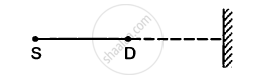Q 28 | Page 354

A source S and a detector D are placed at a distance d apart. A big cardboard is placed at a distance $\sqrt{2}d$ from the source and the detector as shown in figure. The source emits a wave of wavelength = d/2 which is received by the detector after reflection from the cardboard. It is found to be in phase with the direct wave received from the source. By what minimum distance should the cardboard be shifted away so that the reflected wave becomes out of phase with the direct wave?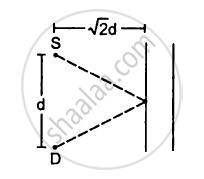Q 29 | Page 353

Two stereo speakers are separated by a distance of 2.40 m. A person stands at a distance of 3.20 m directly in front of one of the speakers as shown in figure. Find the frequencies in the audible range (20-2000 Hz) for which the listener will hear a minimum sound intensity. Speed of sound in air = 320 m s−1.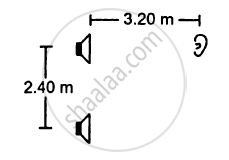Q 30 | Page 354

The two sources of sound, S1 and S2, emitting waves of equal wavelength 20.0 cm, are placed with a separation of 20.0 cm between them. A detector can be moved on a line parallel to S1 S2 and at a distance of 20.0 cm from it. Initially, the detector is equidistant from the two sources. Assuming that the waves emitted by the sources are in detector should be shifted to detect a minimum of sound.

Q 31 | Page 354

Two speakers S1 and S2, driven by the same amplifier, are placed at y = 1.0 m and y = −1.0 m(See figure). The speakers vibrate in phase at 600 Hz. A man stands at a point on the X-axis at a very large distance from the origin and starts moving parallel to the Y-axis. The speed of sound in air is 330 m s−1. (a) At what angle θ will the intensity of sound drop to a minimum for the first time? (b) At what angle will he hear a maximum of sound intensity for the first time? (c) If he continues to walk along the line, how many more can he hear?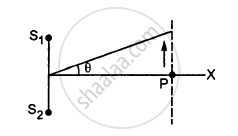Q 32 | Page 354

Three sources of sound S1, S2 and S3 of equal intensity are placed in a straight line with S1S2 = S2S3. At a point P, far away from the sources, the wave coming from S2 is 120° ahead in phase of that from S1. Also, the wave coming from S3 is 120° ahead of that from S2. What would be the resultant intensity of sound at P?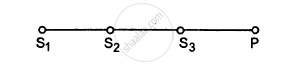Q 33 | Page 354

Two coherent narrow slits emitting sound of wavelength λ in the same phase are placed parallel to each other at a small separation of 2λ. The sound is detected by moving a detector on the screen ∑ at a distance D(>>λ) from the slit S1 as shown in figure. Find the distance x such that the intensity at P is equal to the intensity at O.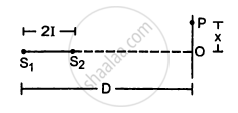Q 34 | Page 354

Figure shown two coherent sources S1 and S2 which emit sound of wavelength λ in phase. The separation between the sources is 3λ. A circular wire of large radius is placed in such way that S1,S2 is at the centre of the wire. Find the angular positions θ on the wire for which constructive interference takes place.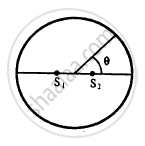Q 35 | Page 355

Two sources of sound S1 and S2 vibrate at same frequency and are in phase. The intensity of sound detected at a point P as shown in the figure is I0. (a) If θ equals 45°, what will be the intensity of sound detected at this point if one of the sources is switched off? (b) What will be the answer of the previous part if θ = 60°?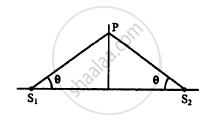Q 36 | Page 355

Find the fundamental, first overtone and second overtone frequencies of an open organ pipe of length 20 cm. Speed of sound in air is 340 ms−1.

Q 37 | Page 355

A closed organ pipe can vibrate at a minimum frequency of 500 Hz. Find the length of the tube. Speed of sound in air = 340 m s−1.

Q 38 | Page 355

In a standing wave pattern in a vibrating air column, nodes are formed at a distance of 4.0 cm. If the speed of sound in air is 328 m s−1, what is the frequency of the source?

Q 39 | Page 355

The separation between a node and the next antinode in a vibrating air column is 25 cm. If the speed of sound in air is 340 m s−1, find the frequency of vibration of the air column.

Q 40 | Page 355

A cylindrical metal tube has a length of 50 cm and is open at both ends. Find the frequencies between 1000 Hz and 2000 Hz at which the air column in the tube can resonate. Speed of sound in air is 340 m s−1.

Q 41 | Page 355

In a resonance column experiment, a tuning fork of frequency 400 Hz is used. The first resonance is observed when the air column has a length of 20.0 cm and the second resonance is observed when the air column has a length of 62.0 cm. (a) Find the speed of sound in air. (b) How much distance above the open end does the pressure node form?

Q 42 | Page 355

The first overtone frequency of a closed organ pipe P1 is equal to the fundamental frequency of a open organ pipe P2. If the length of the pipe P1 is 30 cm, what will be the length of P2?

Q 43 | Page 355

A copper rod of length 1.0 m is clamped at its middle point. Find the frequencies between 20 Hz and 20,000 Hz at which standing longitudinal waves can be set up in the rod. The speed of sound in copper is 3.8 km s−1.

Q 44 | Page 355

Find the greatest length of an organ pipe open at both ends that will have its fundamental frequency in the normal hearing range (20 − 20,000 Hz). Speed of sound in air = 340 m s−1.

Q 45 | Page 355

An open organ pipe has a length of 5 cm. (a) Find the fundamental frequency of vibration of this pipe. (b) What is the highest harmonic of such a tube that is in the audible range? Speed of sound in air is 340 m s−1 and the audible range is 20-20,000 Hz.

Q 46 | Page 355

An electronically driven loudspeaker is placed near the open end of a resonance column apparatus. The length of air column in the tube is 80 cm. The frequency of the loudspeaker can be varied between 20 Hz and 2 kHz. Find the frequencies at which the column will resonate. Speed of sound in air = 320 m s−1.

Q 47 | Page 355

Two successive resonance frequencies in an open organ pipe are 1944 Hz and 2592 Hz. Find the length of the tube. The speed of sound in air is 324 ms−1.

Q 48 | Page 355

A piston is fitted in a cylindrical tube of small cross section with the other end of the tube open. The tube resonates with a tuning fork of frequency 512 Hz. The piston is gradually pulled out of the tube and it is found that a second resonance occurs when the piston is pulled out through a distance of 32.0 cm. Calculate the speed of sound in the air of the tube.

Q 49 | Page 355

A U-tube having unequal arm-lengths has water in it. A tuning fork of frequency 440 Hz can set up the air in the shorter arm in its fundamental mode of vibration and the same tuning fork can set up the air in the longer arm in its first overtone vibration. Find the length of the air columns. Neglect any end effect and assume that the speed of sound in air = 330 m s−1.

Q 50 | Page 355

Consider the situation shown in the figure.The wire which has a mass of 4.00 g oscillates in its second harmonic and sets the air column in the tube into vibrations in its fundamental mode. Assuming that the speed of sound in air is 340 m s−1, find the tension in the wire.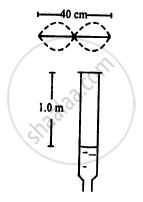Q 51 | Page 355

A 30.0-cm-long wire having a mass of 10.0 g is fixed at the two ends and is vibrated in its fundamental mode. A 50.0-cm-long closed organ pipe, placed with its open end near the wire, is set up into resonance in its fundamental mode by the vibrating wire. Find the tension in the wire. Speed of sound in air = 340 m s−1.

Q 52 | Page 355

Show that if the room temperature changes by a small amount from T to T + ∆T, the fundamental frequency of an organ pipe changes from v to v + ∆v, where $\frac{∆ v}{v} = \frac{1}{2}\frac{∆ T}{T} .$

Q 53 | Page 356

The fundamental frequency of a closed pipe is 293 Hz when the air in it is a temperature of 20°C. What will be its fundamental frequency when the temperature changes to 22°C?

Q 54 | Page 356

A Kundt's tube apparatus has a copper rod of length 1.0 m clamped at 25 cm from one of the ends. The tube contains air in which the speed of sound is 340 m s−1. The powder collects in heaps separated by a distance of 5.0 cm. Find the speed of sound waves in copper.

Q 55 | Page 356

A Kundt's tube apparatus has a steel rod of length 1.0 m clamped at the centre. It is vibrated in its fundamental mode at a frequency of 2600 Hz. The lycopodium powder dispersed in the tube collects into heaps separated by 6.5 cm. Calculate the speed of sound in steel and in air.

Q 56 | Page 356

A source of sound with adjustable frequency produces 2 beats per second with a tuning fork when its frequency is either 476 Hz of 480 Hz. What is the frequency of the tuning fork?

Q 57 | Page 356

A tuning fork produces 4 beats per second with another tuning fork of frequency 256 Hz. The first one is now loaded with a little wax and the beat frequency is found to increase to 6 per second. What was the original frequency of the tuning fork?

Q 58 | Page 356

Calculate the frequency of beats produced in air when two sources of sound are activated, one emitting a wavelength of 32 cm and the other of 32.2 cm. The speed of sound in air is 350 m s−1.

Q 59 | Page 356

A tuning fork of unknown frequency makes 5 beats per second with another tuning fork which can cause a closed organ pipe of length 40 cm to vibrate in its fundamental mode. The beat frequency decreases when the first tuning fork is slightly loaded with wax. Find its original frequency. The speed of sound in air is 320 m s−1.

Q 60 | Page 356

A piano wire A vibrates at a fundamental frequency of 600 Hz. A second identical wire Bproduces 6 beats per second with it when the tension in A is slightly increased. Find the the ratio of the tension in A to the tension in B.

Q 61 | Page 356

A tuning fork of frequency 256 Hz produces 4 beats per second with a wire of length 25 cm vibrating in its fundamental mode. The beat frequency decreases when the length is slightly shortened. What could be the minimum length by which the wire we shortened so that it produces no beats with the tuning fork?

Q 62 | Page 356

A traffic policeman standing on a road sounds a whistle emitting the main frequency of 2.00 kHz. What could be the apparent frequency heard by a scooter-driver approaching the policeman at a speed of 36.0 km h−1? Speed of sound in air = 340 m s−1.

Q 63 | Page 356

The horn of a car emits sound with a dominant frequency of 2400 Hz. What will be the apparent dominant frequency heard by a person standing on the road in front of the car if the car is approaching at 18.0 km h−1? Speed of sound in air = 340 m s−1.

Q 64 | Page 356

A person riding a car moving at 72 km h−1 sound a whistle emitting a wave of frequency 1250 Hz. What frequency will be heard by another person standing on the road (a) in front of the car (b) behind the car? Speed of sound in air = 340 m s−1.

Q 65 | Page 356

A train approaching a platform at a speed of 54 km h−1 sounds a whistle. An observer on the platform finds its frequency to be 1620 Hz. the train passes the platform keeping the whistle on and without slowing down. What frequency will the observer hear after the train has crossed the platform? The speed of sound in air = 332 m s−1.

Q 66 | Page 356

A bat emitting an ultrasonic wave of frequency 4.5 × 104 Hz flies at a speed of 6 m s−1between two parallel walls. Find the fractional heard by the bat and the beat frequencies heard by the bat and the beat frequency between the two. The speed of sound is 330 m s−1.

Q 67 | Page 356

A bullet passes past a person at a speed of 220 m s−1. Find the fractional change in the frequency of the whistling sound heard by the person as the bullet crosses the person. Speed of sound in air = 330 m s−1.

Q 68 | Page 356

Two electric trains run at the same speed of 72 km h−1 along the same track and in the same direction with separation of 2.4 km between them. The two trains simultaneously sound brief whistles. A person is situated at a perpendicular distance of 500 m from the track and is equidistant from the two trains at the instant of the whistling. If both the whistles were at 500 Hz and the speed of sound in air is 340 m s−1, find the frequencies heard by the person.

Q 69 | Page 356

A violin player riding on a slow train plays a 440 Hz note. Another violin player standing near the track plays the same note. When the two are closed by and the train approaches the person on the ground, he hears 4.0 beats per second. The speed of sound in air = 340 m s−1. (a) Calculate the speed of the train. (b) What beat frequency is heard by the player in the train?

Q 70 | Page 356

Two identical tuning forks vibrating at the same frequency 256 Hz are kept fixed at some distance apart. A listener runs between the forks at a speed of 3.0m s−1 so that he approaches one tuning fork and recedes from the other figure. Find the beat frequency observed by the listener. Speed of sound in air = 332 m s−1.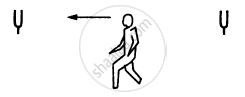Q 71 | Page 356

Figure shows a person standing somewhere in between two identical tuning forks. each vibrating at 512 Hz. If both the tuning forks move towards right a speed of 5.5 m s−1, find the number of beats heard by the listener. Speed of sound in air = 330 m s−1.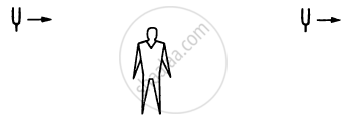Q 72 | Page 356

A small source of sound vibrating at frequency 500 Hz is rotated in a circle of radius 100/π cm at a constant angular speed of 5.0 revolutions per second. A listener situation situates himself in the plane of the circle. Find the minimum and the maximum frequency of the sound observed. Speed of sound in air = 332 m s−1.

Q 73 | Page 356

Two trains are travelling towards each other both at a speed of 90 km h−1. If one of the trains sounds a whistle at 500 Hz, what will be the apparent frequency heard in the other train? Speed of sound in air = 350 m s−1.

Q 74 | Page 356

A traffic policeman sounds a whistle to stop a car-driver approaching towards him. The car-driver does not stop and takes the plea in court that because of the Doppler shift, the frequency of the whistle reaching him might have gone beyond the audible limit of 25 kHz and he did not hear it. Experiments showed that the whistle emits a sound with frequency closed to 16 kHz. Assuming that the claim of the driver is true, how fast was he driving the car? Take the speed of sound in air to be 330 m s−1. Is this speed practical with today's technology?

Q 75 | Page 357

A car moving at 108 km h−1 finds another car in front it going in the same direction at 72 km h−1. The first car sounds a horn that has a dominant frequency of 800 Hz. What will be the apparent frequency heard by the driver in the front car? Speed of sound in air = 330 m s−1.

Q 76 | Page 357

Two submarines are approaching each other in a calm sea. The first submarine travels at a speed of 36 km h−1 and the other at 54 km h−1 relative to the water. The first submarine sends a sound signal (sound waves in water are also called sonar) at a frequency of 2000 Hz. (a) At what frequency is this signal received from the second submarine. At what frequency is this signal received by the first submarine. Take the speed of of the sound wave in water to be 1500 m s−1.

Q 77 | Page 357

A small source of sound oscillates in simple harmonic motion with an amplitude of 17 cm. A detector is placed along the line of motion of the source. The source emits a sound of frequency 800 Hz which travels at a speed of 340 m s−1. If the width of the frequency band detected by the detector is 8 Hz, find the time period of the source.

Q 78 | Page 357

A boy riding on his bike is going towards east at a speed of 4√2 m s−1. At a certain point he produces a sound pulse of frequency 1650 Hz that travels in air at a speed  of 334 m s−1. A second boy stands on the ground 45° south of east from his. Find the frequency of the pulse as received by the second boy.

Q 79 | Page 357

A sound source, fixed at the origin, is continuously emitting sound at a frequency of 660 Hz. The sound travels in air at a speed of 330 m s−1. A listener is moving along the lien x= 336 m at a constant speed of 26 m s−1. Find the frequency of the sound as observed by the listener when he is (a) at y = − 140 m, (b) at y = 0 and (c) at y = 140 m.

Q 80 | Page 357

A train running at 108 km h−1 towards east whistles at a dominant frequency of 500 Hz. Speed of sound in air is 340 m/s. What frequency will a passenger sitting near the open window hear? (b) What frequency will a person standing near the track hear whom the train has just passed? (c) A wind starts blowing towards east at a speed of 36 km h−1. Calculate the frequencies heard by the passenger in the train and by the person standing near the track.

Q 81 | Page 357

A boy riding on a bicycle going at 12 km h−1 towards a vertical wall whistles at his dog on the ground. If the frequency of the whistle is 1600 Hz and the speed of sound in air is 330 m s−1, find (a) the frequency of the whistle as received by the wall (b) the frequency of the reflected whistle as received by the boy.

Q 82 | Page 357

A person standing on a road sends a sound signal to the driver of a car going away from him at a speed of 72 km h−1. The signal travelling at 330 m s−1 in air and having a frequency of 1600 Hz gets reflected from the body of the car and returns. Find the frequency of the reflected signal as heard by the person.

Q 83 | Page 357

A car moves with a speed of 54 km h−1 towards a cliff. The horn of the car emits sound of frequency 400 Hz at a speed of 335 m s−1. (a) Find the wavelength of the sound emitted by the horn in front of the car. (b) Find the wavelength of the wave reflected from the cliff. (c) What frequency does a person sitting in the car hear for the reflected sound wave? (d) How many beats does he hear in 10 seconds between the sound coming directly from the horn and that coming after the reflection?

Q 84 | Page 357

An operator sitting in his base camp sends a sound signal of frequency 400 Hz. The signal is reflected back from a car moving towards him. The frequency of the reflected sound is found to be 410 Hz. Find the speed of the car. Speed of sound in air = 324 m s−1

Q 85 | Page 357

Figure shows a source of sound moving along X-axis at a speed of 22 m s−1continuously emitting a sound of frequency 2.0 kHz which travels in air at a speed of 330 m s−1. A listener Q stands on the Y-axis at a distance of 330 m from the origin. At t = 0, the sources crosses the origin P. (a) When does the sound emitted from the source at P reach the listener Q? (b) What will be the frequency heard by the listener at this instant? (c) Where will the source be at this instant?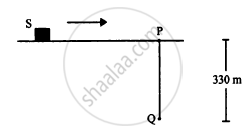Q 86 | Page 357

A source emitting sound at frequency 4000 Hz, is moving along the Y-axis with a speed of 22 m s−1. A listener is situated on the ground at the position (660 m, 0). Find the frequency of the sound received by the listener at the instant the source crosses the origin. Speed of sound in air = 330 m s−1.

Q 87 | Page 357

A source of sound emitting a 1200 Hz note travels along a straight line at a speed of 170 m s−1. A detector is placed at a distance 200 m from the line of motion of the source. (a) Find the frequency of sound receive by the detector at the instant when the source gets closest to it. (b) Find the distance between the source and the detector at the instant in detects the frequency 1200 Hz. Velocity of sound in air = 340 m s−1.

Q 88 | Page 357

A small source of sound S of frequency 500 Hz is attached to the end of a light string and is whirled in a vertical circle of radius 1.6 m. The string just remains tight when the source is at the highest point. (a) An observer is located in the same vertical plane at a large distance and at the same height as the centre of the circle. The speed of sound in air = 330 m s−1 and = 10 m s−2. Find the maximum frequency heard by the observer. (b) An observer is situated at a large distance vertically above the centre of the circle. Find the frequency heard by the observer corresponding to the sound emitted by the source when it is at the same height as the centre.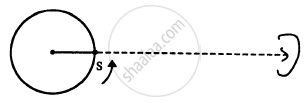Q 89 | Page 357

A source emitting a sound of frequency v is placed at a large distance from an observer. The source starts moving towards the observer with a uniform acceleration a. Find the frequency heard by the observer corresponding to the wave emitted just after the source starts. The speed of sound in the medium is v.

## Chapter 16: Sound Waves

#### HC Verma Concept of Physics Part-1 (2018-2019 Session) by H.C Verma## HC Verma solutions for Class 11 Physics chapter 16 - Sound Waves

HC Verma solutions for Class 11 Physics chapter 16 (Sound Waves) include all questions with solution and detail explanation. This will clear students doubts about any question and improve application skills while preparing for board exams. The detailed, step-by-step solutions will help you understand the concepts better and clear your confusions, if any. Shaalaa.com has the CBSE Concept of Physics Part-1 (2018-2019 Session) by H.C Verma solutions in a manner that help students grasp basic concepts better and faster.

Further, we at Shaalaa.com provide such solutions so that students can prepare for written exams. HC Verma textbook solutions can be a core help for self-study and acts as a perfect self-help guidance for students.

Concepts covered in Class 11 Physics chapter 16 Sound Waves are Speed of Wave Motion, Reflection of Transverse and Longitudinal Waves, Displacement Relation for a Progressive Wave, The Speed of a Travelling Wave, Principle of Superposition of Waves,, Introduction of Reflection of Waves, Standing Waves and Normal Modes, Beats, Doppler Effect, Wave Motion.

Using HC Verma Class 11 solutions Sound Waves exercise by students are an easy way to prepare for the exams, as they involve solutions arranged chapter-wise also page wise. The questions involved in HC Verma Solutions are important questions that can be asked in the final exam. Maximum students of CBSE Class 11 prefer HC Verma Textbook Solutions to score more in exam.

Get the free view of chapter 16 Sound Waves Class 11 extra questions for Physics and can use Shaalaa.com to keep it handy for your exam preparation

S